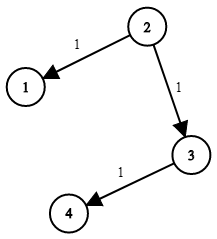# [LeetCode] 743. Network Delay Time 网络延迟时间

There are N network nodes, labelled 1 to N.

Given times, a list of travel times as directededges times[i] = (u, v, w), where u is the source node, v is the target node, and w is the time it takes for a signal to travel from source to target.

Now, we send a signal from a certain node K. How long will it take for all nodes to receive the signal? If it is impossible, return -1.

Example 1:Input: times = [[2,1,1],[2,3,1],[3,4,1]], N = 4, K = 2
Output: 2


Note:

1. N will be in the range [1, 100].
2. K will be in the range [1, N].
3. The length of times will be in the range [1, 6000].
4. All edges times[i] = (u, v, w) will have 1 <= u, v <= N and 0 <= w <= 100.

class Solution {
public:
int networkDelayTime(vector<vector<int>>& times, int N, int K) {
int res = 0;
vector<vector<int>> edges(101, vector<int>(101, -1));
queue<int> q{{K}};
vector<int> dist(N + 1, INT_MAX);
dist[K] = 0;
for (auto e : times) edges[e][e] = e;
while (!q.empty()) {
unordered_set<int> visited;
for (int i = q.size(); i > 0; --i) {
int u = q.front(); q.pop();
for (int v = 1; v <= 100; ++v) {
if (edges[u][v] != -1 && dist[u] + edges[u][v] < dist[v]) {
if (!visited.count(v)) {
visited.insert(v);
q.push(v);
}
dist[v] = dist[u] + edges[u][v];
}
}
}
}
for (int i = 1; i <= N; ++i) {
res = max(res, dist[i]);
}
return res == INT_MAX ? -1 : res;
}
};

class Solution {
public:
int networkDelayTime(vector<vector<int>>& times, int N, int K) {
int res = 0;
vector<int> dist(N + 1, INT_MAX);
dist[K] = 0;
for (int i = 1; i < N; ++i) {
for (auto e : times) {
int u = e, v = e, w = e;
if (dist[u] != INT_MAX && dist[v] > dist[u] + w) {
dist[v] = dist[u] + w;
}
}
}
for (int i = 1; i <= N; ++i) {
res = max(res, dist[i]);
}
return res == INT_MAX ? -1 : res;
}
};

class Solution {
public:
int networkDelayTime(vector<vector<int>>& times, int N, int K) {
int res = 0;
unordered_map<int, vector<pair<int, int>>> edges;
vector<int> dist(N + 1, INT_MAX);
queue<int> q{{K}};
dist[K] = 0;
for (auto e : times) edges[e].push_back({e, e});
while (!q.empty()) {
int u = q.front(); q.pop();
unordered_set<int> visited;
for (auto e : edges[u]) {
int v = e.first, w = e.second;
if (dist[u] != INT_MAX && dist[u] + w < dist[v]) {
dist[v] = dist[u] + w;
if (visited.count(v)) continue;
visited.insert(v);
q.push(v);
}
}
}
for (int i = 1; i <= N; ++i) {
res = max(res, dist[i]);
}
return res == INT_MAX ? -1 : res;
}
};

Github 同步地址：

https://github.com/grandyang/leetcode/issues/743

https://leetcode.com/problems/network-delay-time/description/

https://leetcode.com/problems/network-delay-time/discuss/109982/C++-Bellman-Ford

https://leetcode.com/problems/network-delay-time/discuss/109968/Simple-JAVA-Djikstra's-(PriorityQueue-optimized)-Solution-with-explanation

LeetCode All in One 题目讲解汇总(持续更新中...)

posted @ 2018-01-12 23:52  Grandyang  阅读(7842)  评论(8编辑  收藏